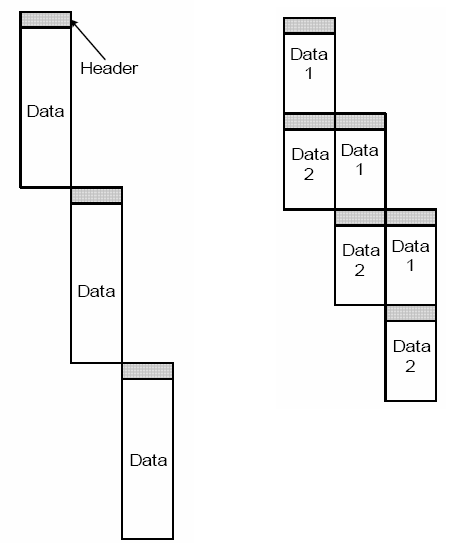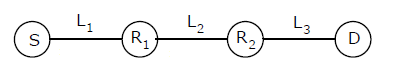Related Articles
Computer Networks | Set 6
• Difficulty Level : Hard
• Last Updated : 27 Mar, 2017

Following questions have been asked in GATE CS 2005 exam.

1) An organization has a class B network and wishes to form subnets for 64 departments. The subnet mask would be:
(a) 255.255.0.0
(b) 255.255.64.0
(c) 255.255.128.0
(d) 255.255.252.0

Answer (d)
The size of network ID is 16 bit in class B networks. So bits after 16th bit must be used to create 64 departments. Total 6 bits are needed to identify 64 different departments. Therefore, subnet mask will be 255.255.252.0.

2) In a packet switching network, packets are routed from source to destination along a single path having two intermediate nodes. If the message size is 24 bytes and each packet contains a header of 3 bytes, then the optimum packet size is:
(a) 4
(b) 6
(c) 7
(d) 9

Answer (d)
Dividing a message into packets may decrease the transmission time due to parallelism as shown in the following figure.But after a certain limit reducing the packet size may increase the transmission time also.

Following figure shows the situation given in question.Let transmission time to transfer 1 byte for all nodes be t. The first packet will take time = (packet size)*3*t. After the first packet reaches the destination, remaining packets will take time equal to (packet size)*t due to parallelism.

```If we use 4 bytes as packet size, there will be 24 packets
Total Transmission time = Time taken by first packet +
Time taken by remaining packets
= 3*4*t + 23*4*t = 104t

If we use 6 bytes as packet size, there will be 8 packets
Total Transmission time = 3*6*t + 7*6*t = 60t

If we use 7 bytes as packet size, there will be 6 packets
Total Transmission time = 3*7*t + 5*7*t = 56t

If we use 9 bytes as packet size, there will be 4 packets
Total Transmission time = 3*9*t + 3*9*t = 54t
```

3) Suppose the round trip propagation delay for a 10 Mbps Ethernet having 48-bit jamming signal is 46.4 ms. The minimum frame size is:
(a) 94
(b) 416
(c) 464
(d) 512

Answer (c)
Transmission Speed = 10Mbps.
Round trip propagation delay = 46.4 ms
The minimum frame size = (Round Trip Propagation Delay) * (Transmission Speed) = 10*(10^6)*46.4*(10^-3) = 464 * 10^3 = 464 Kbit

The concept behind the above formula is collision detection. Consider a situation where a node A wants to send a frame to another node B. When Node A begins transmitting, the signal must propagate the network length. In the worst-case collision scenario, Node B begins to transmit just before the signal for Node A’s frame reaches it. The collision signal of Node A and Node B’s frame must travel back to Node A for Node A to detect that a collision has occurred.
The time it takes for a signal to propagate from one end of the network to the other is known as the propagation delay. In this worst-case collision scenario, the time that it takes for Node A to detect that its frame has been collided with is twice the propagation delay. Node A’s frame must travel all the way to Node B, and then the collision signal must travel all the way from Node B back to Node A. This time is known as the slot time. An Ethernet node must be transmitting a frame for the slot time for a collision with that frame to be detected. This is the reason for the minimum Ethernet frame size.

Please see GATE Corner for all previous year paper/solutions/explanations, syllabus, important dates, notes, etc.

Please write comments if you find any of the answers/explanations incorrect, or you want to share more information about the topics discussed above

Attention reader! Don’t stop learning now. Get hold of all the important CS Theory concepts for SDE interviews with the CS Theory Course at a student-friendly price and become industry ready.

My Personal Notes arrow_drop_up